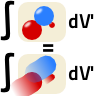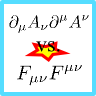# Relativistic Electrodynamics

Before we come to the actual problems below, let us elaborate a little on relativistic notation and the conventions we may use. Electrodynamics is a Lorentz invariant theory. This means that certain Lorentz coordinate transformations leave the theory invariant, i.e. their observables or equations of motion. These transformations show that electric and magnetic fields can be transformed into each other. The simplest example is an electron at rest. It only has an electric field. However, if the coordinate system is moving, we find that this electron corresponds to some current also causing a magnetic field.

So, the Lorentz invariance of electrodynamics makes it possible to combine electric and magnetic fields. Before we come to this point, we may define the four-current and potentials in SI-units as$\begin{eqnarray*} j^{\mu}&=&\left(c\rho,\mathbf{j}\right)\ \mathrm{and}\\A^{\mu}&=&\left(\frac{\phi}{c},\mathbf{A}\right)\ .\end{eqnarray*}$In this notation, the wave equation in vacuum may be written as$\begin{eqnarray*} \sum_{\alpha=0}^{3}\partial_{\alpha}\partial^{\alpha}A^{\mu}&=&-\mu_{0}j^{\mu}\ . \end{eqnarray*}$Furthermore it is very handy to use the Einstein summation convention: any equal index appearing up and down is summed over every possible element, i.e. $$\sum_{\alpha}\partial_{\alpha}\partial^{\alpha}\equiv\partial_{\alpha}\partial^{\alpha}$$. Indices are “pulled” up and down with the metric $$\eta=\eta_{\mu\nu}dx^{\mu}dx^{\nu}$$. In a cartesian coordinate system the metric obeys the form$\begin{eqnarray*} \eta_{\mu\nu}&=&\left(\begin{array}{cccc}-1 & 0 & 0 & 0\\0 & 1 & 0 & 0\\0 & 0 & 1 & 0\\0 & 0 & 0 & 1\end{array}\right)\ :\\A_{\mu}&=&\eta_{\mu\nu}A^{\nu}=\left(-\frac{\phi}{c},\mathbf{A}\right)\ .\end{eqnarray*}$We already encountered the concept of the metric for a surface in Geodesic Movement of a Charge on a Surface with Electric Field as a measure for distances. Here, we would have to think a little further what our notion of distance actually means. There is even another ambiguity one has to mention here. If one does not set the speed of light to unity, $$c=1$$, which is often done, there should appear a $$-c^{2}$$ in the $$\eta_{00}$$ component. If one however sets $$dx^{0}=cdt$$, we get rid of this little inconsistency. Note that$\begin{eqnarray*} \eta^{\mu\alpha}\eta_{\alpha\nu}&=&\delta_{\nu}^{\mu}\ . \end{eqnarray*}$The fields itself come into play as the derivatives of the potentials. The relativistic generalization of electric and magnetic fields is the electromagnetic field tensor (explicit in cartesian coordinates):$\begin{eqnarray*} F_{\mu\nu} = \partial_{\nu}A_{\mu}-\partial_{\mu}A_{\nu}=\left(\begin{array}{cccc}0 & -\frac{E_{x}}{c} & -\frac{E_{y}}{c} & -\frac{E_{z}}{c}\\\frac{E_{x}}{c} & 0 & B_{z} & -B_{y}\\\frac{E_{y}}{c} & -B_{z} & 0 & B_{x}\\\frac{E_{z}}{c} & B_{y} & -B_{x} & 0\end{array}\right)_{\mathrm{cart.}}. \end{eqnarray*}$Then, Maxwell's equations in vacuum can be cast in the rather condensed form$\begin{eqnarray*} \partial_{\gamma}F_{\mu\nu}+\partial_{\nu}F_{\gamma\mu}+\partial_{\mu}F_{\nu\gamma}&=&0\ ,\\\partial_{\nu}F^{\mu\nu}&=&\mu_{0}j^{\mu}\ . \end{eqnarray*}$Do you see what the Lagrangian $$\mathcal{L}_{2}$$ looks like in terms of electric and magnetic fields?

There is a lot more to be said about Lorentz transformations, their physical effects like length contraction and time dilation and so on. If you want to learn more about these concepts and phenomena, you may enjoy introductory texts on general relativity. In the first chapters you will always find a sufficient outline of special relativity and a lot of interesting examples why it is so important to see our world as (at least) four-dimensional entity. As a starting point you may consider S.M. Carroll's “Lecture Notes on General Relativity”.

But now, lets get our hands down dirty and solve some problems:

## How the Gauge Invariance of the Action implies Charge ConservationUsually, gauge transformations are used to simplify certain calculations. In this problem, we want to use them to derive charge conservation from the invariance of the action $$S$$.

## Lorentz Invariance of the Four-PotentialLorentz invariance is at the core of any (special) relativistic theory. Sometimes, however, we encounter expressions that seem to transform in a wrong way. This is especially true for the four-potential calculated from the retarded four-current. Find out why the four-potential is indeed Lorentz invariant!

## An Alternative LagrangianUsing the useful index notation of special relativity we will find that one may use different Lagrangians to describe relativistic electrodynamics. This problem will make you familiar with the basic rules needed for practical calculations.